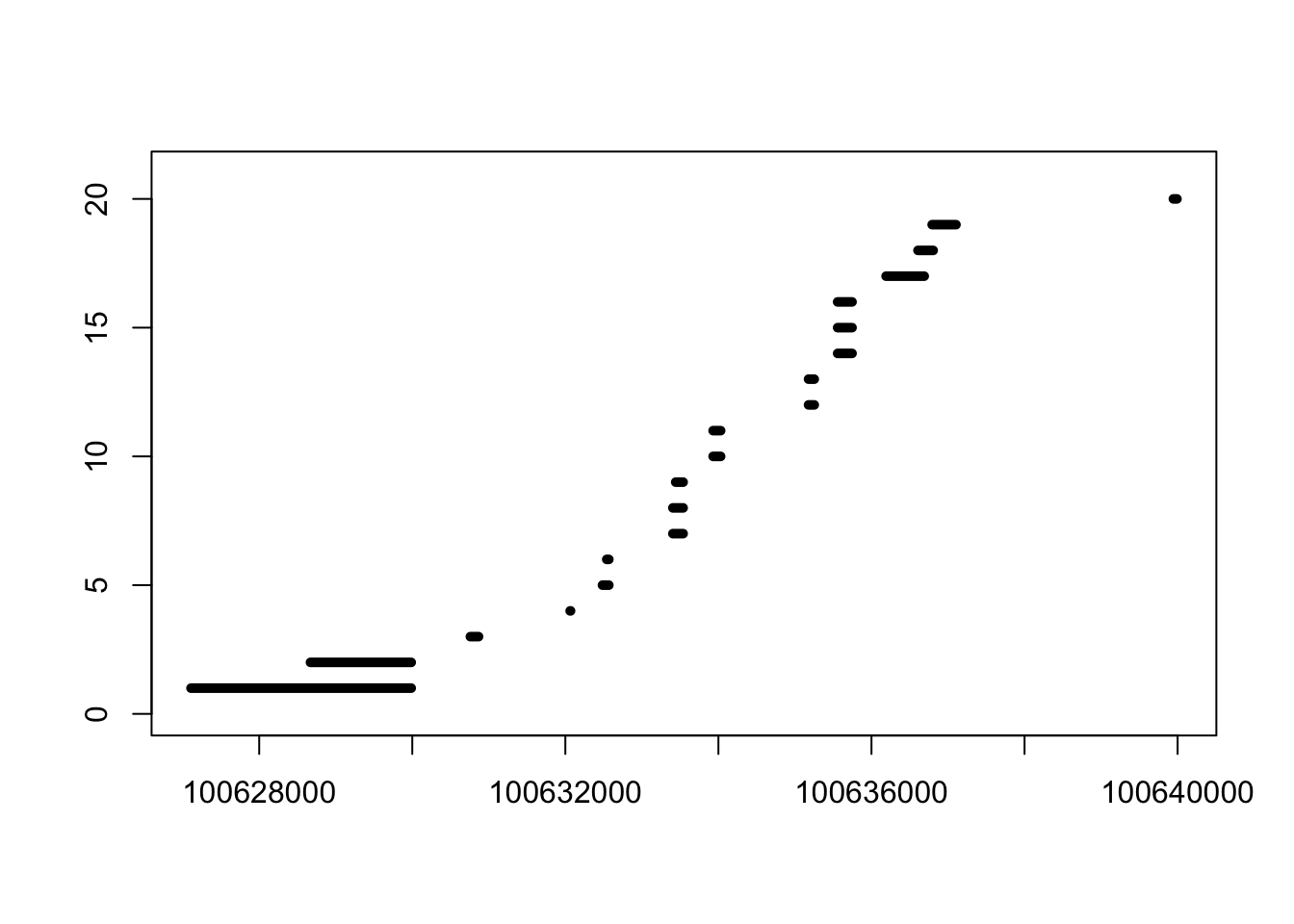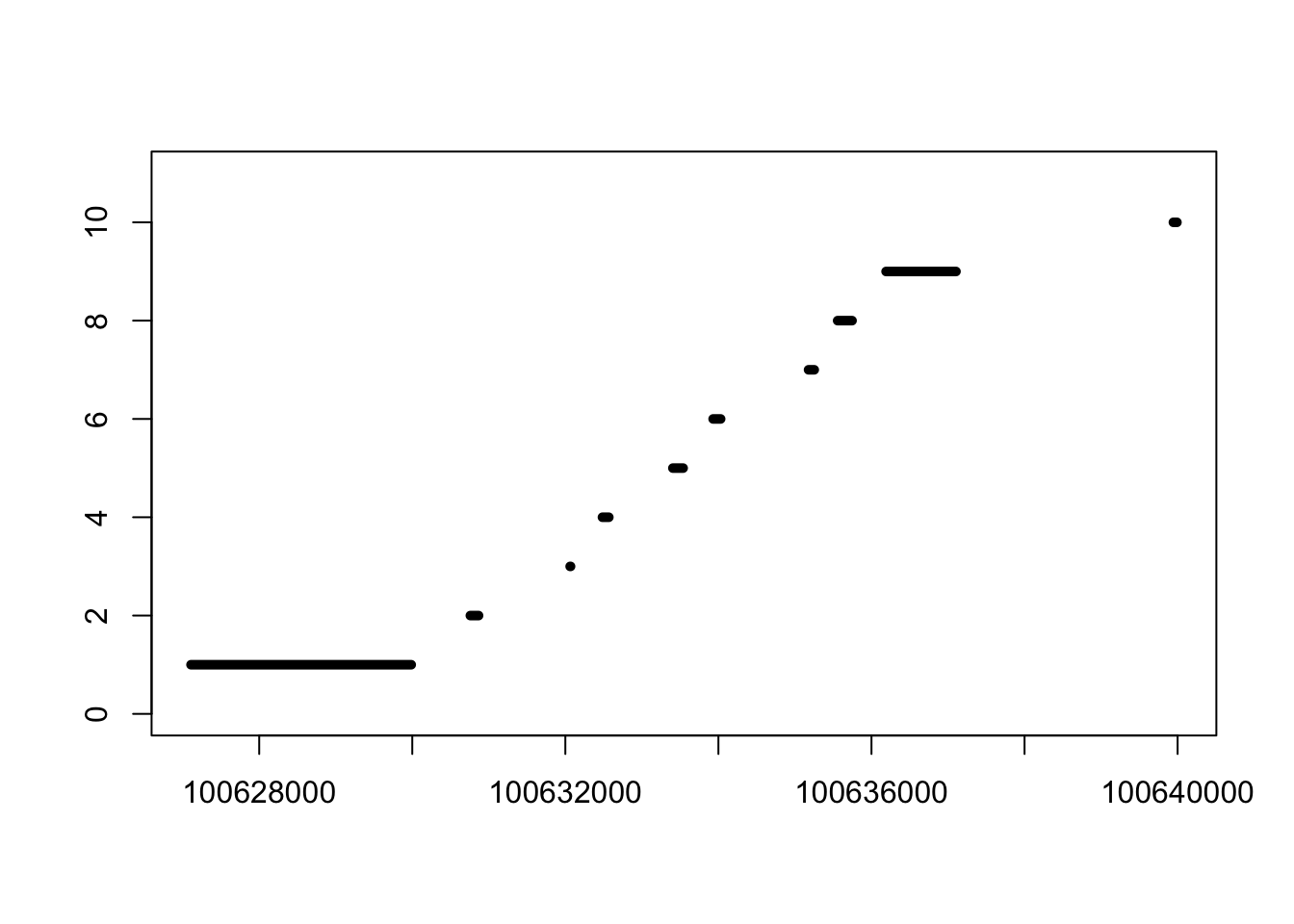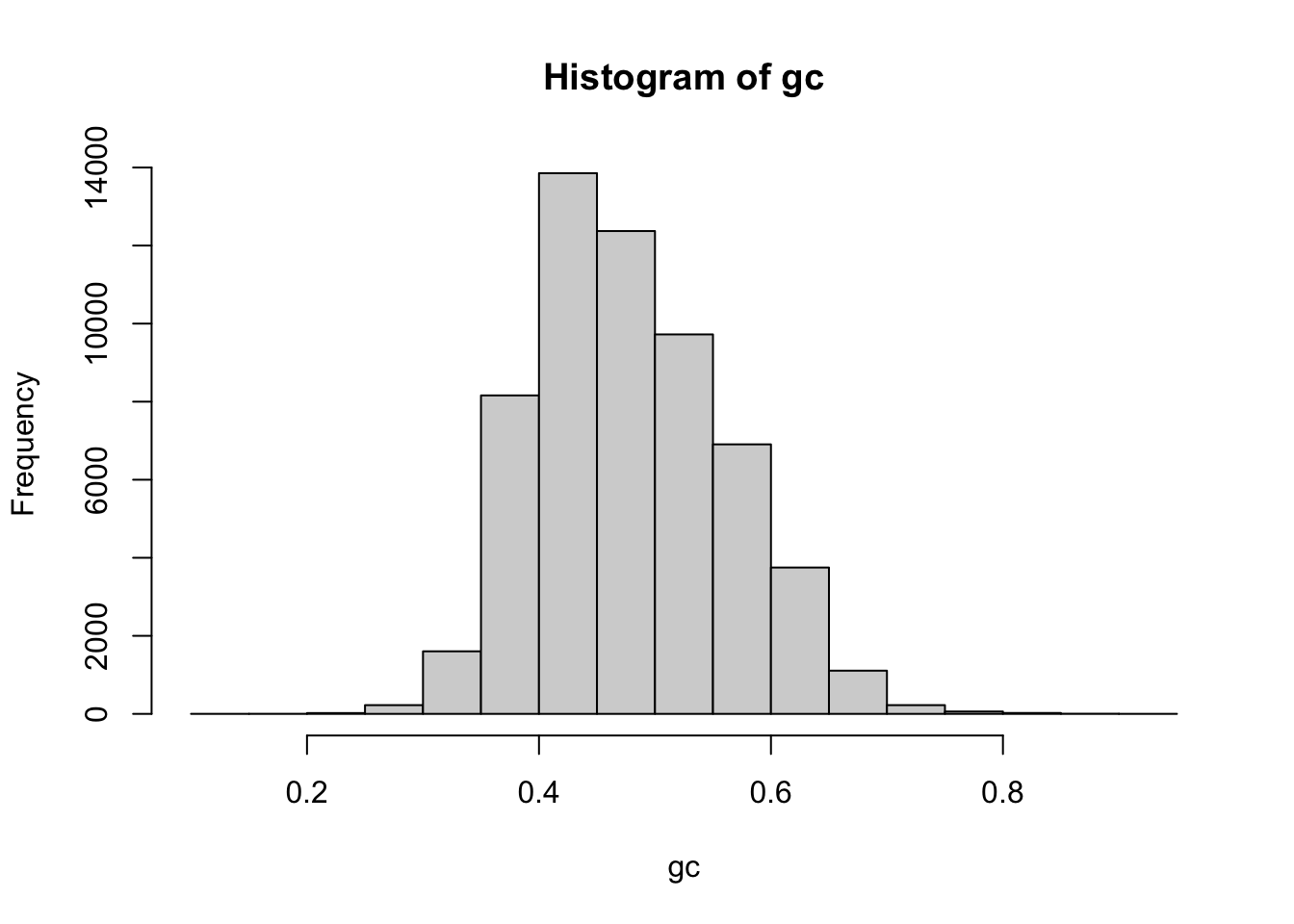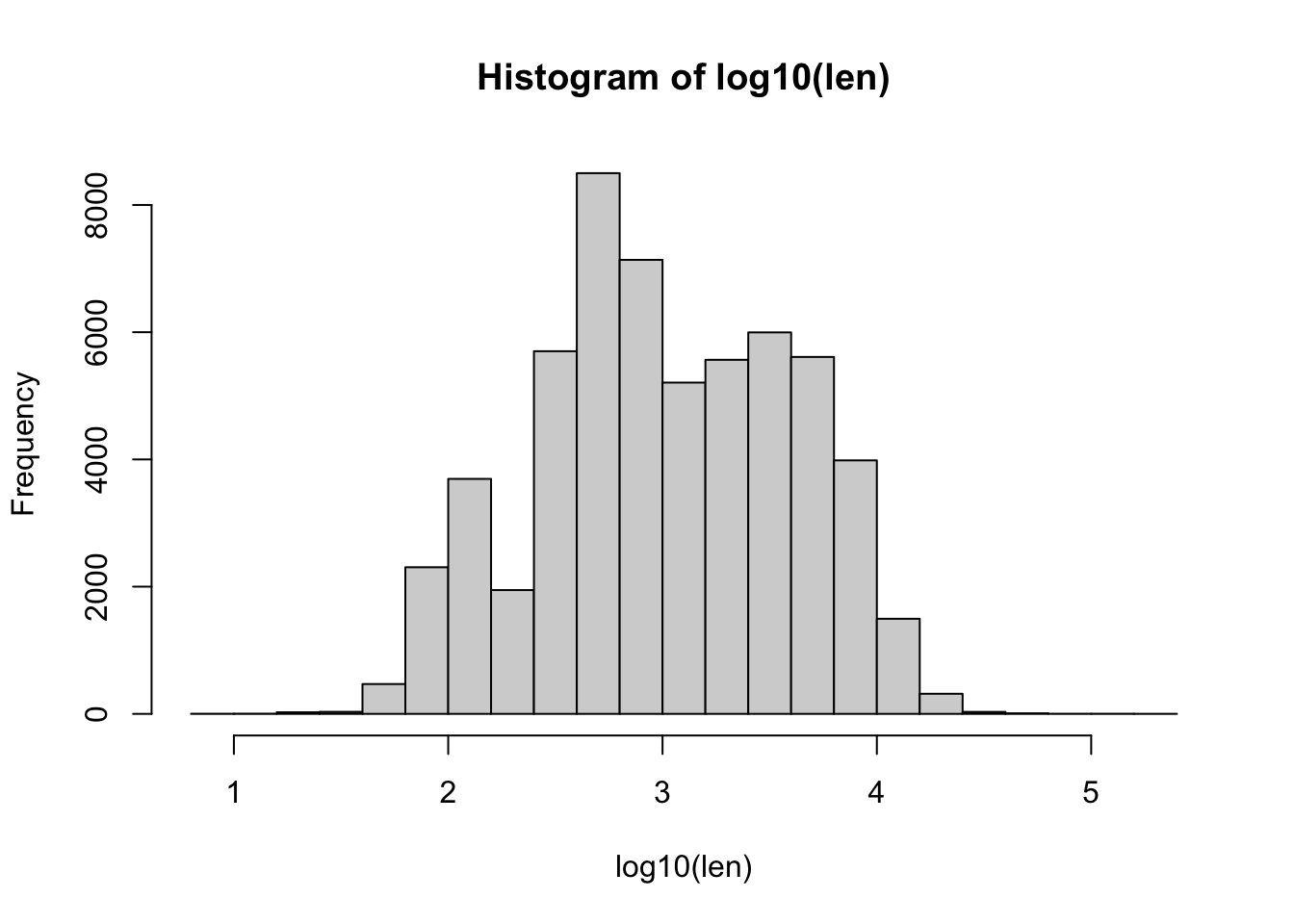Previously, we looked at distances between samples in high-throughput sequencing experiments, exploring sequencing depth as a technical artifact, the effect of various transformation on stabilizing variance, and PCA and hierarchical clustering as methods for ordination of samples.

In distances, we noticed many genes where we see differences in measurements across the sequencing center, and in hclust, we saw high level clustering of samples by sequencing center, when we focused on a single human population. These technical differences in high-throughput measurements are often referred to as “batch effects”, a term which encompasses any kind of technical artifact due to the lab or time at which a sample was processed. Note that the time of sample preparation, the batch, can have its own unique technical distortion on measurements, just as much as a sample from a different sequencing center.

Two references which give a general outline of batch effects in sequencing data are:

It is critical, if you are involved in the design of a high-throughput experiment, that the biological conditions are not confounded with the sample preparation batches, instead to use either a block or randomized design. Block designs are easy, simply ensuring that each sample preparation batch contains each of the biological conditions of interest, so that the batch effects can be isolated apart from the biological differences between samples.

Here I will show the origin of batch effects across lab can sometimes be isolated to batch-specific distortions related to the DNA sequence of the genes. As described in the second link above, a step in nearly all high-throughput experiments is to amplify the DNA fragments using PCR. As PCR is an exponential process of copying molecules, it is very sensitive to slight variations, and results in distortions of measurements which are specific to the particular place and time when the samples were processed: the batch.

We start again with the GEUVADIS RNA-seq samples prepared in distances.

``````library(DESeq2)

As typical, though we conceptually have a simple task to perform, many steps are needed just to get the covariates that we need. Here our simple task is to model the counts on covariates like the genes’ lengths and sequence content. However, it will take many steps to obtain and summarize the sequence content into a single number.

A critical summary of DNA sequence is the GC content, which is the fraction of G’s and C’s out of the total sequence. Note that, because G pairs with C, and C with G, the GC content of a sequence and it’s complement is identical. GC content varies along the genome, for biological reasons. Different organisms have very different GC content of their genomes. But we care about GC content also for a technical reason: because the GC content of a piece of DNA can make it difficulty to amplify. We will see this below.

In order to get the GC content of each gene, we start by downloading the information about the genes used by recount2 for quantification, which was version 25 of the Gencode genes. We download this file from the following link (37 Mb).

``library(GenomicFeatures)``

We need to import this gene information from a GTF (gene transfer format) file, and turn it into a TxDb. We then save the database, so we can skip the `makeTxDbFromGFF` step in the future. Note that a warning message about `The "phase" metadata column contains non-NA values`, and another one about closing connection are both OK.

``````txdb <- makeTxDbFromGFF("gencode.v25.annotation.gtf.gz")
saveDb(txdb, file="gencode.sqlite")``````

We load the TxDb and extract all the exons, grouped by gene. We check that we have the same gene names in `ebg` and in `dds`.

``````txdb <- loadDb("gencode.sqlite")
ebg <- exonsBy(txdb, by="gene")
``````##  "ENSG00000000003.14" "ENSG00000000005.5"  "ENSG00000000419.12" "ENSG00000000457.13"
##  "ENSG00000000460.16" "ENSG00000000938.12"``````
``table(names(ebg) %in% rownames(dds))``
``````##
##  TRUE
## 58037``````
``table(rownames(dds) %in% names(ebg))``
``````##
##  TRUE
## 58037``````

Note that the exons in `ebg` contain redundant sequence. We can see this by plotting the ranges for a given gene. Note that, after running `reduce`, we remove all redundant sequence in `ebg` for a given gene.

``````e <- ebg[]
library(rafalib)
plotRanges <- function(e) {
l <- length(e)
r <- ranges(range(e))
nullplot(start(r), end(r), 0, l+1)
segments(start(e), 1:l, end(e), 1:l, lwd=5)
}
plotRanges(e)````````plotRanges(reduce(e))``Now we put the reduced exons in correct order (in this case they are already in correct order), and we store them as `rowRanges` of the dataset.

``````exons <- reduce(ebg)
exons <- exons[ rownames(dds) ]
rowRanges(dds) <- exons``````

# Calculate GC content and length of reduced exons

Now we extract the exonic sequence for every gene, using the `extractTranscriptSeqs` function.

``````library(BSgenome.Hsapiens.UCSC.hg38)
dna <- extractTranscriptSeqs(Hsapiens, rowRanges(dds))``````

We then calculate the GC content (ratio of G or C to total basepairs) with `letterFrequency`, and save this as a metadata column `gc`. We also save the total number of basepairs to a metadata column `len`.

``````mcols(dds)\$gc <- as.numeric(letterFrequency(dna, "GC", as.prob=TRUE))
mcols(dds)\$len <- sum(width(rowRanges(dds)))``````
``with(mcols(dds), hist(gc))````with(mcols(dds), hist(log10(len)))``We know have all the covariates we need for modeling how the counts vary by GC content. We can make simple plots to see if we see a dependence. Note that, outside of GC content of .35-.65, we see very few large counts, although there do appear to be genes with this GC content. It is very difficult to amplify the fragments of cDNA from these genes, and so they are often missing from high-throughput sequencing experiments like RNA-seq.

``````plot(mcols(dds)\$gc, log10(counts(dds)[,1]+1), cex=.1)
abline(v=c(.35,.65))``````